# Classical orthogonal polynomials

The general term for Jacobi polynomials; Hermite polynomials; and Laguerre polynomials. These systems of orthogonal polynomials have the following properties in common:

1) The weight function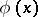on the interval of orthogonality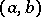satisfies the Pearson differential equationwhere the following conditions hold at the end points of the interval of orthogonality:2) The polynomial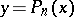of ordersatisfies the differential equation3) The Rodrigues formula holds:whereis a normalizing coefficient.

4) Derivatives of classical orthogonal polynomials are also classical orthogonal polynomials and are orthogonal on the same interval of orthogonality, generally speaking with a different weight.

5) For the generating functionthe representationholds, where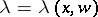is the root of the quadratic equation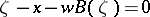that is nearest tofor small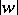.

Only the three systems of orthogonal polynomials mentioned satisfy these properties; for systems obtained from these three by linear transformations of the independent variable these properties also hold.

In the generalized Rodrigues formula, the normalizing coefficient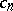is usually chosen by three different methods with the aim of either obtaining orthonormal polynomials, orthogonal polynomials with unit leading coefficient or so-called standardized orthogonal polynomials, for which the main formulas have the simplest form and which prove to be most convenient in applications.

The classical orthogonal polynomials are the eigen functions of certain eigen value problems for equations of Sturm–Liouville type. In these problems, each system of orthogonal polynomials (Jacobi polynomials, Hermite polynomials and Laguerre polynomials) is the unique sequence of solutions of the corresponding system of equations (see ).

Particular cases of the classical orthogonal polynomials are defined by the following choices of weight function and interval of orthogonality:

1) The Jacobi polynomials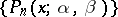are orthogonal on the interval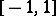with weight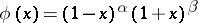, where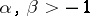. In particular, the casegives the ultraspherical polynomials or Gegenbauer polynomials. The Legendre polynomials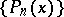correspond to the values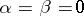and are orthogonal onwith weight. If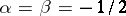, that is,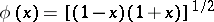, then one obtains the Chebyshev polynomials of the first kind,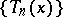, while for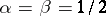, the Chebyshev polynomials of the second kind,, are obtained.

2) The Hermite polynomialsare orthogonal on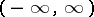with weight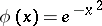.

3) The Laguerre polynomialsare orthogonal onwith weight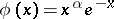, where.

See also the references to Orthogonal polynomials.